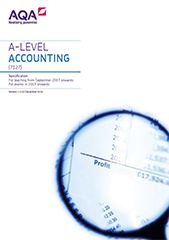# Appendix: accounting ratios

Students will be required to know the formulae for the following accounting ratios.

Ratio

Formula

Capital gearing

Current ratio

Expressed as x:1

Dividend cover

Dividend yield

Earnings per share

Note: for a group the ratio is:

Profit attributable to ordinary shareholders of the parent company (Profit after tax in pence)/Number of issued ordinary shares.

Expenses in relation to revenue

Gross profit margin

Interest cover

Liquid capital ratio (acid test ratio)

Expressed as x:1

Markup

Price earnings

Profit in relation to revenue

Rate of inventory turnover

Average inventory: normally the average of the opening and closing inventories.

Rate of inventory turnover (days)

Return on capital employed (sole trader)

Capital employed is capital + non-current liabilities (either opening or closing capital could be used in the calculation).

Return on capital employed (limited company)

Capital employed is Equity + non-current liabilities (either opening or closing figure for equity could be used in the calculation).